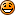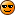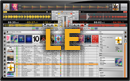## Cross MIDI output mapping

### Re: Cross MIDI output mapping

Basically you are trying to use 2 different commands: 1) Fx "select" to switch led on. 2) Fx "on/off" to switch led off. In your "On" mapping you are using an integer ("7"). In your "off" mapping you are using a Logic ("false"). I suggest changing the alias to "select" and the "false" to a number... if thats possible without lighting up another pad. Alternatively using the alias "fx selectandtoggle" to do the switching on & off. This is just a suggestion - I'm no mapping guruacemcPosts: 237
Joined: 04 Mar 2013, 19:46
Location: South Africa

### Re: Cross MIDI output mapping

acemc wrote:Basically you are trying to use 2 different commands: 1) Fx "select" to switch led on. 2) Fx "on/off" to switch led off. In your "On" mapping you are using an integer ("7"). In your "off" mapping you are using a Logic ("false"). I suggest changing the alias to "select" and the "false" to a number... if thats possible without lighting up another pad. Alternatively using the alias "fx selectandtoggle" to do the switching on & off. This is just a suggestion - I'm no mapping guruCan't use a logic statement like tru or false to turn off an LED.
fx selectandtoggle expects a numeric value to perform the action in the system and the value can be given to specify that once the value is received turn on LED #999. To turn of the same LED I would have to specify a logic condition true or false which i tried to do with (Fx)[0x1].OnOff (Logic) because when you toggle the pad a second time the effect is turned off which should set the output of (Fx)[0x1].OnOff (Logic) to false.FigDJPosts: 1351
Joined: 22 Apr 2012, 16:03
Location: Odenton, MD

### Re: Cross MIDI output mapping

Yep, you're correct. I just took a look at my mappings, from what I can see, it's pretty much the same as yours. Ie: It should work, unless the "fxselectandtoggle" is holding just the one led on.
Perhaps you can set the "fxselectandtoggle" to actually toggle between 2 numbers, like 1 for on & 0 for off?
MEMBER OF:c00l People MV SocietyacemcPosts: 237
Joined: 04 Mar 2013, 19:46
Location: South Africa

### Re: Cross MIDI output mapping

<mapping>
<control alias="fam(Fx)[0x1].SelectAndToggle" value="7"/>
<MIDI type="Note" parameter="0x9" channel="0x1" value="0"/>
<MIDI type="Note" parameter="0xb" channel="0x1" value="0"/>
<MIDI type="Note" parameter="0xd" channel="0x1" value="127"/>
<MIDI type="Note" parameter="0xf" channel="0x1" value="0"/>
<MIDI type="Note" parameter="0x1" channel="0x1" value="0"/>
<MIDI type="Note" parameter="0x3" channel="0x1" value="0"/>
<MIDI type="Note" parameter="0x5" channel="0x1" value="0"/>
<MIDI type="Note" parameter="0x7" channel="0x1" value="0"/>
</mapping>

<mapping>
<control alias="fam(Fx)[0x1].SelectAndToggle" value="0"/>
<MIDI type="Note" parameter="0x9" channel="0x1" value="0"/>
<MIDI type="Note" parameter="0xb" channel="0x1" value="0"/>
<MIDI type="Note" parameter="0xd" channel="0x1" value="0"/>
<MIDI type="Note" parameter="0xf" channel="0x1" value="0"/>
<MIDI type="Note" parameter="0x1" channel="0x1" value="0"/>
<MIDI type="Note" parameter="0x3" channel="0x1" value="0"/>
<MIDI type="Note" parameter="0x5" channel="0x1" value="0"/>
<MIDI type="Note" parameter="0x7" channel="0x1" value="0"/>
</mapping>

There are 15 values from 0 to 14 and there are only 14 fx, so one of the value is surely for OFF, i guess it is 0.Support@MixVibes

### Re: Cross MIDI output mapping

Support@MixVibes wrote:
There are 15 values from 0 to 14 and there are only 14 fx, so one of the value is surely for OFF, i guess it is 0.

I see what you are saying, however my specific challenge is as follows.

Counting from top to bottom and left to right.
Pads 1-8 are for other purposes, that leaves pads 9 to 16 free to map.
I picked the 8 effects that I like the most to map

4/Delay/9
6/X Phaser/10
7/Flanger/11
8/Jet/12
9/Transform/13
11/Crush/14
12/Bliss/15
14/Roll/16

When a pad is pressed the particular pad is lit and all other are not by using the code below.
(Example for Flanger on pad #11)
<mapping>
<control alias="fam(Fx)[0x1].SelectAndToggle" value="7"/>
<MIDI type="Note" parameter="0x9" channel="0x1" value="0"/>
<MIDI type="Note" parameter="0xb" channel="0x1" value="0"/>
<MIDI type="Note" parameter="0xd" channel="0x1" value="127"/>
<MIDI type="Note" parameter="0xf" channel="0x1" value="0"/>
<MIDI type="Note" parameter="0x1" channel="0x1" value="0"/>
<MIDI type="Note" parameter="0x3" channel="0x1" value="0"/>
<MIDI type="Note" parameter="0x5" channel="0x1" value="0"/>
<MIDI type="Note" parameter="0x7" channel="0x1" value="0"/>
</mapping>

The specific pad has to be pressed a second time to turn the effect off. This actions do toggle the output from

In&Out fam(Fx)[0x1].OnOff (Logic)

correcly, however the selection is still value 7.

The LED flickers momentarily because

<mapping>
<control alias="fam(Fx)[0x1].OnOff" value="false"/>
<MIDI type="Note" parameter="0x9" channel="0x1" value="0"/>
<MIDI type="Note" parameter="0xb" channel="0x1" value="0"/>
<MIDI type="Note" parameter="0xd" channel="0x1" value="0"/>
<MIDI type="Note" parameter="0xf" channel="0x1" value="0"/>
<MIDI type="Note" parameter="0x1" channel="0x1" value="0"/>
<MIDI type="Note" parameter="0x3" channel="0x1" value="0"/>
<MIDI type="Note" parameter="0x5" channel="0x1" value="0"/>
<MIDI type="Note" parameter="0x7" channel="0x1" value="0"/>
</mapping>

turns the LED off but
<control alias="fam(Fx)[0x1].SelectAndToggle" value="7"/>
is still selected.

If the second time the pad is pressed the selection would reset to 0, then all the above logic would work.

<mapping>
<control alias="fam(Fx)[0x1].SelectAndToggle" value="0"/>
<MIDI type="Note" parameter="0x9" channel="0x1" value="0"/>
<MIDI type="Note" parameter="0xb" channel="0x1" value="0"/>
<MIDI type="Note" parameter="0xd" channel="0x1" value="0"/>
<MIDI type="Note" parameter="0xf" channel="0x1" value="0"/>
<MIDI type="Note" parameter="0x1" channel="0x1" value="0"/>
<MIDI type="Note" parameter="0x3" channel="0x1" value="0"/>
<MIDI type="Note" parameter="0x5" channel="0x1" value="0"/>
<MIDI type="Note" parameter="0x7" channel="0x1" value="0"/>
</mapping>

would only work if I assign assign another button to select the value 0 which defeats the purpose of just pressing one button.

In short my dode doesn't work because

press pad 2nd time=selection stays the same + effect is turned off.

My code would work if

press pad 2nd time=selection resets to 0 + effect is turned off.

I appreciate the support, however, given the conditions below,

press pad 2nd time=selection stays the same + effect is turned off.

Your suggestion will only work by pressing a different button to reset selection to 0.

press pad 2nd time=selection stays the same + effect is turned off.
press another buton or pad to change selection to 0

I hope this topic is as interesting to you as it is to me because I see this things as small but challenging puzzles that can be solved.FigDJPosts: 1351
Joined: 22 Apr 2012, 16:03
Location: Odenton, MD

### Re: Cross MIDI output mapping

I did a quick test and the fx on-off false that switch off everything works.

Code: Select all
`      <output-mappings>        <mapping>          <control alias="fam(Fx)[0x1].SelectAndToggle" value="4"/>          <MIDI type="Note" parameter="0xa" channel="0x1" value="127"/>          <MIDI type="Note" parameter="0xb" channel="0x1" value="0"/>          <MIDI type="Note" parameter="0xc" channel="0x1" value="0"/>        </mapping>        <mapping>          <control alias="fam(Fx)[0x1].SelectAndToggle" value="6"/>          <MIDI type="Note" parameter="0xa" channel="0x1" value="0"/>          <MIDI type="Note" parameter="0xb" channel="0x1" value="127"/>          <MIDI type="Note" parameter="0xc" channel="0x1" value="0"/>        </mapping>        <mapping>          <control alias="fam(Fx)[0x1].SelectAndToggle" value="7"/>          <MIDI type="Note" parameter="0xa" channel="0x1" value="0"/>          <MIDI type="Note" parameter="0xb" channel="0x1" value="0"/>          <MIDI type="Note" parameter="0xc" channel="0x1" value="127"/>        </mapping>        <mapping>          <control alias="fam(Fx)[0x1].OnOff" value="false"/>          <MIDI type="Note" parameter="0xa" channel="0x1" value="0"/>          <MIDI type="Note" parameter="0xb" channel="0x1" value="0"/>          <MIDI type="Note" parameter="0xc" channel="0x1" value="0"/>        </mapping>      </output-mappings>`Support@MixVibes

### Re: Cross MIDI output mapping

Support@MixVibes wrote:I did a quick test and the fx on-off false that switch off everything works.

Thanks the response, when I get home I am going to try aggain the way you suggest. Perhaps when I tried the 1st time I missed something or typed something wrong. It wouldn't the 1st time nor it will be the last that a little code detail bites me.

Once I try it and test I''ll get back here with results.

ThanksFigDJPosts: 1351
Joined: 22 Apr 2012, 16:03
Location: Odenton, MD

### Re: Cross MIDI output mapping

Support@MixVibes wrote:I did a quick test and the fx on-off false that switch off everything works.

Heey, thank you so much. I had tried your same way of coding and it didn't work (that was before we started the conversation).

Then we talked (over here) and it made me go back and re examine the code.

In between mapping statements I found a couple of special characters that I must have typed by mistake from the beginning and were messing up the entire thing.

After fixing that and doing a very close scrutiny of the code (which is the same way you suggested and was my original intend) everything works like a charm.

Thanks you so much for keeping me in the right path.
The Mixvibes team is awesome.FigDJPosts: 1351
Joined: 22 Apr 2012, 16:03
Location: Odenton, MD

### Re: Cross MIDI output mapping

Can you share with us your final mapping? Just zip it here.UncleVibesPosts: 10254
Joined: 22 Sep 2003, 13:36

### Re: Cross MIDI output mapping

has anyone had any success with mapping jog LEDs? i've tried using delayA/delayB on a denon sc2900, without success.
apparently, this seems to be the current holy grail for quite a few denon 2900 users.kugmoPosts: 92
Joined: 04 Jul 2007, 10:31
Location: Cebu City, Philippines

PreviousNext

### Who is online

Users browsing this forum: No registered users and 4 guests

### Board index